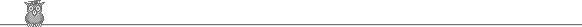### 14th Putnam 1954Problem B6

If x is a positive rational, show that we can find distinct positive integers a1, a2, ... , an such that x = ∑ 1/ai.

Solution

Hard.

Rather surprisingly, the simplest possible algorithm - the greedy algorithm - works: take an to be the smallest integer not already chosen so that the sum does not exceed x. This terminates after a finite number of steps.

Note that the numbers involved can be quite large even in simple cases. For example: 4 = 1/1 + 1/2 + 1/3 + ... + 1/30 + 1/200 + 1/77706 + 1/16532869712 + 1/3230579689970657935732 + 1/36802906522516375115639735990520502954652700

The key idea is that the numerator of the difference gets smaller each time and hence the process must terminate.

First, we have to get the difference under 1. So take m such that 1 + 1/2 + ... + 1/m ≤ x < 1 + 1/2 + ... + 1/(m+1). This is possible since the harmonic series diverges. If we have equality, then we are home. If not then the difference x - (1 + 1/2 + ... + 1/m) < 1/(m+1).

Let the difference x - (1/a1 + 1/a2 + ... + 1/an) be dn = rn/sn (where rn and sn are integers).

So we may assume that we have picked a1, ... , an and that dn < 1/(an + 1). We now take an+1 so that 1/an+1 ≤ dn < 1/(an+1 - 1). Now dn+1 = dn - 1/an+1 = (rnan+1 - sn)/(snan+1). This expression may not be in lowest terms, but it is certainly sufficient to show that (rnan+1 - sn) < rn or dnan+1 - 1 < dn. But that is true since dn(an+1 - 1) < 1. Finally, we notice that dn+1 < 1/(an+1 + 1) is equivalent to dn - 1/an+1 < 1/(an+1 + 1), which is true since 1/(an+1 - 1) - 1/an+1 < 1/(an+1 + 1), since an+1 > 1.